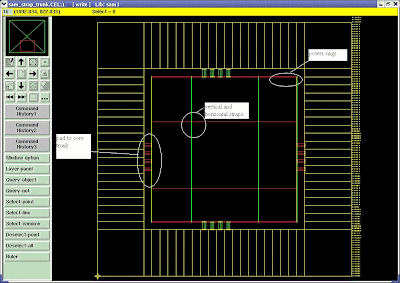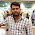20 October 2007

Power Planning

There are two types of power planning and management. They are core cell power management and I/O cell power management. In former one VDD and VSS power rings are formed around the core and macro. In addition to this straps and trunks are created for macros as per the power requirement. In the later one, power rings are formed for I/O cells and trunks are constructed between core power ring and power pads. Top to bottom approach is used for the power analysis of flatten design while bottom up approach is suitable for macros.

The power information can be obtained from the front end design. The synthesis tool reports static power information. Dynamic power can be calculated using Value Change Dump (VCD) or Switching Activity Interchange Format (SAIF) file in conjunction with RTL description and test bench. Exhaustive test coverage is required for efficient calculation of peak power. This methodology is depicted in Figure (1).

For the hierarchical design budgeting has to be carried out in front end. Power is calculated from each block of the design. Astro works on flattened netlist. Hence here top to bottom approach can be used. JupiterXT can work on hierarchical designs. Hence bottom up approach for power analysis can be used with JupiterXT. IR drops are not found in floor planning stage. In placement stage rails are get connected with power rings, straps, trunks. Now IR drops comes into picture and improper design of power can lead to large IR drops and core may not get sufficient power.Figure (1) Power Planning methodology

Below are the calculations for flattened design of the SAMM. Only static power reported by the Synthesis tool (Design Compiler) is used instead of dynamic power.

• The number of the core power pad required for each side of the chip

= total core power / [number of side*core voltage*maximum allowable current for a I/O pad]

= 236.2068mW/ [4 * 1.08 V * 24mA] (Considering design SAMM)

= 2.278

~ 2

Therefore for each side of the chip 2 power pads (2 VDD and 2 VSS) are added.

• Total dynamic core current (mA)

= total dynamic core power / core voltage

= 236.2068mW / 1.08V

= 218.71 mA

• Core PG ring width
= (Total dynamic core current)/ (No. of sides * maximum current density of the metal layer used (Jmax) for PG ring)
=218.71 mA/(4*49.5 mA/µm)
~1.1 µm
~2 µm
• Pad to core trunk width (µm)

= total dynamic core current / number of sides * Jmax where Jmax is the maximum current density of metal layer used

= 218.71 mA / [4 * 49.5 mA/µm]

= 1.104596 µm

Hence pad to trunk width is kept as 2µm.

Using below mentioned equations we can calculate vertical and horizontal strap width and required number of straps for each macro.

• Block current:

Iblock= Pblock / Vddcore

• Current supply from each side of the block:

Itop=Ibottom= { Iblock *[Wblock / (Wblock +Hblock)] }/2

Ileft=Iright= { Iblock *[Hblock / (Wblock +Hblock)] }/2

• Power strap width based on EM:

Wstrap_vertical =Itop / Jmetal

Wstrap_horizontal =Ileft / Jmetal

• Power strap width based on IR:

Wstrap_vertical >= [ Itop * Roe * Hblock ] / 0.1 * VDD

Wstrap_horizontal >= [ Ileft * Roe * Wblock ] / 0.1 * VDD

• Refresh width:

Wrefresh_vertical =3 * routing pitch +minimum width of metal (M4)

Wrefresh_horizontal =3 * routing pitch +minimum width of metal (M3)

• Refresh number

Nrefresh_vertical = max (Wstrap_vertical ) / Wrefresh_vertical

Nrefresh_horizontal = max (Wstrap_horizontal ) / Wrefresh_horizontal

• Refresh spacing

Srefresh_vertical = Wblock / Nrefresh_vertical

Srefresh_horizontal = Hblock / Nrefresh_horizontalFigure (2) Showing core power ring, Straps and Trunks

Related Articles

1.What about PG ring width?

2.core PG ring width= (total core current)/ (No. of sides * maximum current density of the metal layer used for PG ring)

3.I think that formulas for Current supply from each side of the block: should be

Itop=Ibottom= { Iblock *[Wblock / (Wblock +Hblock)] }/2

Ileft=Iright= { Iblock *[Hblock / (Wblock +Hblock)] }/2

Could you please recheck?

4.I think that formulas for Current supply from each side of the block: should be

Itop=Ibottom= { Iblock *[Wblock / (Wblock +Hblock)] }/2

Ileft=Iright= { Iblock *[Hblock / (Wblock +Hblock)] }/2

Could you please recheck?

5.what is Roe here

6.What is Roe & routing pitch and from where we get these values???

1.Hi, Roe is the sheet resistance of the metal layer used for the routing, that is,the resistivity rho divided by the thickness of the routing layer

7.how to draw PG mesh accurately?

8.where we get core voltage? its get from .lib library or not

9.Can any one tell.,
steps to calculate powerplanning with formulaes in Vlsi chip design.

10.fro where we get maximum current density of metal value?

11.why it is using dynamic power to calculate pg, not static + dynamic? thank you.

1.dynamic power is the maximum consumed in the chip.

12.some people say our power planning was a push down structure, what does this mean?